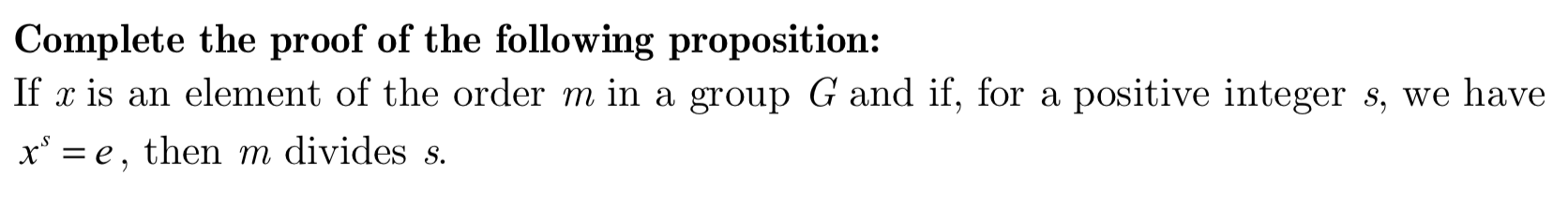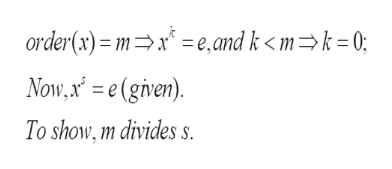# Complete the proof of the following proposition:If x is an element of the order m in a group G and if, for a positive integer s, we havexe then m divides s.

Question
1 viewshelp_outlineImage TranscriptioncloseComplete the proof of the following proposition: If x is an element of the order m in a group G and if, for a positive integer s, we have xe then m divides s. fullscreen
check_circle

Step 1

To prove the required divisibility property of the order m of an element

Step 2

By definition, the order of an element x in a group G is the SMALLEST positive integer m such that x^m =e.

Step 3

Problem statement (here we a...help_outlineImage Transcriptioncloseorder(x) = mx = e,and k < m=k = 0; Now,x =e(given) To show, m divides fullscreen

### Want to see the full answer?

See Solution

#### Want to see this answer and more?

Solutions are written by subject experts who are available 24/7. Questions are typically answered within 1 hour.*

See Solution
*Response times may vary by subject and question.
Tagged in

### Math